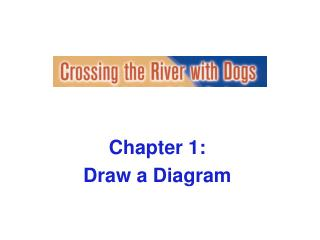DownloadDownload PresentationChapter 1: Draw a Diagram

# Chapter 1: Draw a Diagram

Download Presentation## Chapter 1: Draw a Diagram

- - - - - - - - - - - - - - - - - - - - - - - - - - - E N D - - - - - - - - - - - - - - - - - - - - - - - - - - -
##### Presentation Transcript

1. Chapter 1: Draw a Diagram

2. Curly used a shovel to dig his own swimming pool. He figured he needed a pool because digging it was hard work and he could use it to cool off after working on it all day. He also planned to build a rectangular concrete deck around the pool that would be 6 feet wide at all points. The pool is rectangular and measures 14 feet by 40 feet. What is the area of the deck?

3. Try ideas. 52 feet + 26 feet + 52 feet + 26 feet = 156 feet 156 feet x 6 feet = 936 square feet Counts each corner twice!

4. Keep drawing diagrams. Two lengths: 40 ft x 6 ft x 2 = 480 sq ft Two widths: 14 ft x 6 ft x 2 = 168 sq ft Four corners: 6 ft x 6 ft x 4 = 144 sq ft Total 792 sq ft

5. And another . . . 52 ft x 6 ft = 312 sq ft 312 sq ft x 2 = 624 sq ft for extended lengths 14 ft x 6 ft = 84 sq ft 84 sq ft x 2 = 168 sq ft for widths Total = 624 sq ft + 168 sq ft = 792 sq ft

6. And another . . . Area of entire figure = 52 ft x 26 ft = 1352 sq ft Area of pool alone = 40 ft x 14 ft = 560 sq ft Area of deck = 1352 – 560 = 792 sq ft

7. Strategy: Draw a diagram • Do not hesitate to draw. • You don’t have to be an artist to draw a useful diagram. • If you can visualize it, draw a diagram!